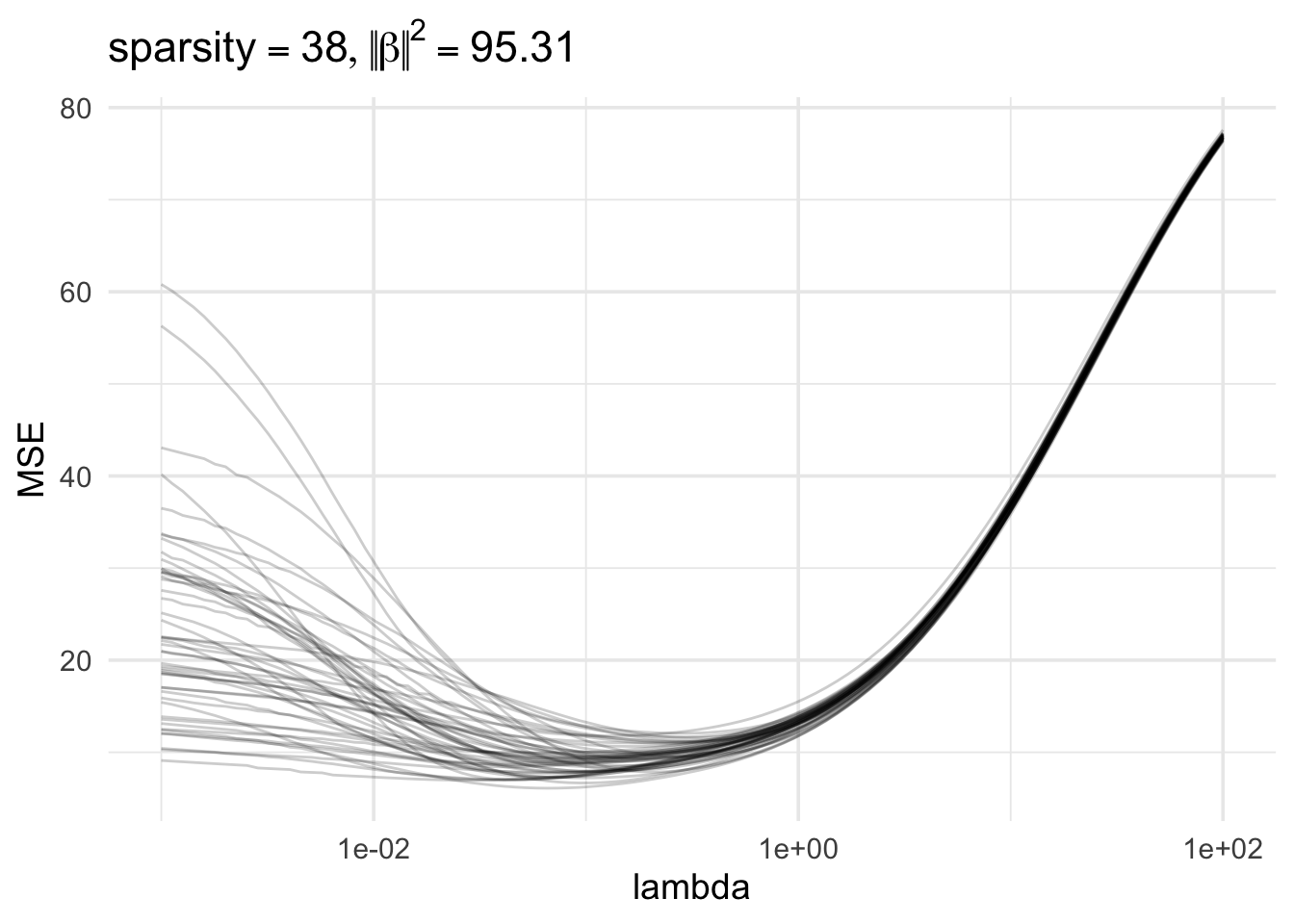## Lasso simulations

### Functions to simulate data

The functions below do the following.

1. Simulate a sparse high-dimensional linear model $\mathbf y = \mathbf X \beta + \varepsilon, \text{ for } \varepsilon \sim N(0, \sigma^2 I)$
2. Fit ridge regression on a grid of $$\lambda$$ values
3. Iterate over multiple realizations of $$\varepsilon$$
4. Plot the MSE of estimated coefficients as a function of $$\lambda$$, with one line for each iterate

$\text{MSE}(\hat \beta_\text{ridge}(\lambda))$

In a real dataset we would only see one of these lines, but the simulation gives us a sense of how the performance could vary depending on the randomness of the noise in the linear model. (Optional: change the functions so you can also specify the noise level and see how larger noise variance affect the results)

instance <- function(X, L, n, p, beta, mu) {
Y <- mu + rnorm(n)
y <- Y - mean(Y)
# Fit model with glmnet
ridge_fit <- glmnet(X, y, standardize = TRUE, intercept = FALSE, alpha = 0, lambda = L)
# Extract estimate coefficients
beta_mat <- coef(ridge_fit)[-1, ]
# Compute MSE using true beta
MSEs <- apply(beta_mat, 2, function(beta_hat) sum((beta_hat - beta)^2))
out <- as.numeric(MSEs)
names(out) <- L
out
}
high_dim_MSE_MC <- function(n, p, instances = 10) {
## Generating signal
# A random sparse coefficient vector
beta <- rnorm(p) * rpois(p, 1) * rbinom(p, 1, .5)
# Fixed design matrix and mean
X <- matrix(rnorm(n*p), nrow = n)
mu <- X %*% beta
# Lambda grid
L <- exp(seq(
from = log(max(n, p, sum(beta^2))),
to = log(1e-3), length.out = n))
## Generate many noise instances
# tidyverse version of replicate()
output <- tibble(inst = 1:instances) %>%
mutate(MSEs = map(inst, ~instance(X, L, n, p, beta, mu)))
## Transform output to long data.frame
out_df <- output %>%
unnest_longer("MSEs") %>%
mutate(MSEs_id = as.numeric(MSEs_id))
names(out_df) <- c("instance", "MSE", "lambda")
## Plot results
out_df %>%
ggplot(aes(lambda, MSE)) +
ggtitle(bquote(
list(sparsity == .(sum(beta != 0)),
abs(abs(beta))^2 == .(round(sum(beta^2), 2)))
)) +
geom_line(aes(group = factor(instance)),
alpha = .2,
show.legend = FALSE) +
scale_x_log10()
}

Delete the set.seed() line and run the function a few times to see the results.

set.seed(123456789) # delete this line
high_dim_MSE_MC(n = 100, p = 100, instances = 50)### Performance of the lasso

Copy/paste and modify the previous functions and use them to experiment with the lasso

• Choose some metric to plot, like MSE of $$\hat \beta_\text{lasso}(\lambda)$$, out of sample prediction accuracy, or true/false positive rates of selected variables.

• Experiment with the parameters, $$n$$, $$p$$, sparsity, or making the design matrix $$X$$ have correlated columns, to see how the performance changes